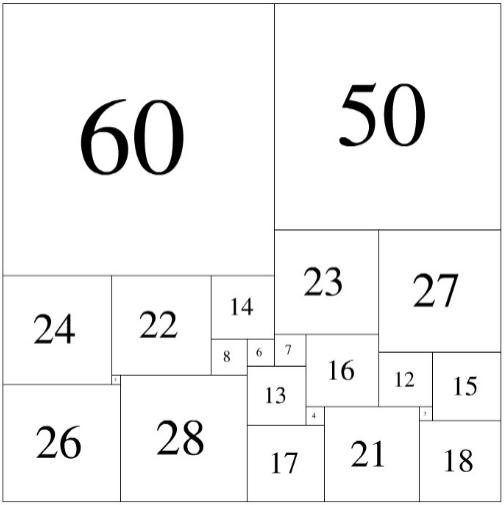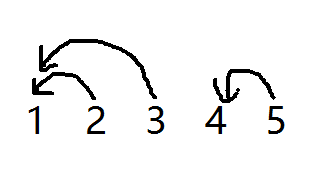37 篇文章 1 订阅

# 1. 积分之迷

第二笔：4个A + 10个B + 1个C，共返积分：420

【答案】：105

【分析】：

3A+7B+C=315       (1)

4A+10B+C=420      (2)

2*(1)-(2)得：

2A+4B+C=210       (3)

(2)-(1)得：

A+3B=105            (4)

(3)-(4)得：

A+B+C=105

# 2. 完美正方形

21 22 23 24 26 27 28 50 60 如【图1.png】那样组合，就是一种解法。此时， 紧贴上边沿的是：60 50

22 24 26 30 31 33 35 36 41 46 47 50 52 61 如果告诉你该方案紧贴着上边沿的是从左到右依次为：47
46 61， 你能计算出紧贴着下边沿的是哪几个正方形吗？ 请提交紧贴着下边沿的正方形的边长，从左到右，用空格分开。# 3. 关联账户

b,c间有关联，则认为a,c间也有关联。 对于调查范围内的n个账户（编号0到n-1），警方已知道m条因转账引起的直接关联。

#include <stdio.h>
#define N 100

int connected(int* m, int p, int q)
{
return m[p]==m[q]? 1 : 0;
}

void link(int* m, int p, int q)
{
int i;
if(connected(m,p,q)) return;
int pID = m[p];
int qID = m[q];
for(i=0; i<N; i++) _____________________________________;  //填空位置
}

int main()
{
int m[N];
int i;
for(i=0; i<N; i++) m[i] = i; //初始状态，每个节点自成一个连通域

printf("%d ", connected(m,4,7));
printf("%d ", connected(m,4,5));
printf("%d ", connected(m,7,9));
printf("%d ", connected(m,9,2));
return 0;
}

【答案】：if(  connected(m,pID,i)  )     m[i]=qID ;

【解析】：当前要连接的两个点，让其中一个的所有关联点，去等于另一个点的老大。# 4.密文搜索

main函数需要返回0 注意: 只使用ANSI C/ANSI C++ 标准，不要调用依赖于编译环境或操作系统的特殊函数。 注意:

【解析】；

0对应a，1对应b。。。

1、对于输入的长串，每个长度为8的区间打一个表，以十进制存储。

2、对于每一个输入的短串，转化为10进制数字

3、对长串打好的表扫描一遍，找一找有多少个相等的短串即可（我用了二分查找法）

【代码】：

#include<stdio.h>
#include<string.h>
#include<algorithm>
using namespace std;
typedef long long ll;
char str[1024*1025],s;
ll a[1024*1025];//存每个区间的串的10进制形式
ll cont;//存每个串的10进制形式
int n;
ll zhuan(char ss[])//把长度为8的26进制字符串转化为10进制数
{
ll ans=0;
char s1;
memcpy(s1,ss,9);
sort(s1,s1+8);//注意排序
for(int i=0;i<8;i++)
ans = ans*26 + (s1[i]-'a');
return ans;
}
void find(ll c[],int l,int r,ll num,int &cnt)
{
if(l==r){
cnt += c[l]==num;
return;
}
int mid=(l+r)/2;
if(c[mid]>=num)
find(c,l,mid,num,cnt);
if(c[mid+1]<=num)
find(c,mid+1,r,num,cnt);
}
int main()
{
scanf("%s%d",str,&n);
for(int i=0;str[i+7];i++)
{
a[i]=zhuan(str+i);
printf("a：%lld\n",a[i]);
}
for(int k=0;k<n;k++)
{
scanf("%s",s);
sort(s,s+8);
cont[k]=zhuan(s);
printf("count:  %lld\n",cont[k]);
}
sort(cont,cont+n);
ll ans=0;
for(int i=0;str[i+7];i++)//对每个区间找相等的(二分查找)
{
int cnt=0;
find(cont,0,n-1,a[i],cnt);//在cont中找a[i]的个数
ans+=cnt;
}
printf("%lld\n",ans);
return 0;
}

# 5. 居民集会

(p1<=p2<=p3<=p4=L),使得村内所有人的路程开销和最小。 【输入格式】 输入的第一行包含两个整数n,
L，分别表示蓝桥村的家庭数和公路长度。 接下来n行，每行两个整数di, ti，分别表示第i户家庭距离公路起点的距离和家庭中的人数。
【输出格式】 输出一行，包含一个整数，表示村内所有人路程的开销和。 【样例输入】 6 10 1 3 2 2 4 5 5 20 6 5 8 7
【样例输出】 18 【样例说明】 在距起点2, 5, 8, 10这4个地方集会，6个家庭需要的走的距离分别为1, 0, 1, 0, 2,
0，总的路程开销为1*3+0*2+1*5+0*20+2*5+0*7=18。 【数据规模与约定】 对于10%的评测数据，1<=n<=300。

1<=n<=100000，1<=L<=1000000，0<=di<=L，di<=di+1，0<=ti<=1000000。 资源约定：

# 6.模型染色

【输入格式】 输入的第一行包含三个整数n, m, k， 分别表示小宏的玩具上的端点数、边数和小宏可能使用的颜色数。端点从1到n编号。

【样例输出】 6 【样例说明】 令(a, b,
c)表示第一个端点染成a，第二个端点染成b，第三个端点染成c，则下面6种本质不同的染色：(1, 1, 1), (1, 1, 2), (1,
2, 1), (1, 2, 2), (2, 1, 2), (2, 2, 2)。 而(2, 1, 1)与(1, 1, 2)是本质相同的，(2,
2, 1)与(2, 1, 2)是本质相同的。 【数据规模与约定】 对于20%的评测数据，1<=n<=5， 1<=k<=2。

1<=k<=30。 资源约定： 峰值内存消耗 < 512M CPU消耗 < 5000ms

main函数需要返回0 注意: 只使用ANSI C/ANSI C++ 标准，不要调用依赖于编译环境或操作系统的特殊函数。 注意:

05-101035
05-0767305-13104
04-193171
04-151771
11-01101
03-286051
05-21561
05-201万+
05-21809
07-171万+
09-26232
04-211005
01-30161
05-2023
05-222017
10-16174
05-294万+
07-171万+点击重新获取扫码支付余额充值